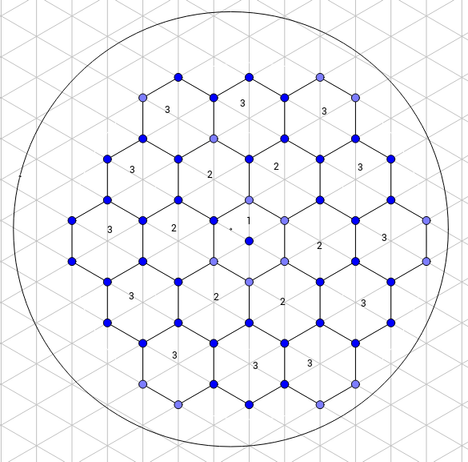# Hexagonal Packingjackal_1586The Tough Summer Spar 201...
Limits 1s, 512 MB

Bees are very hard working insects and they are also very good at geometry. In order to minimize the unusable space, they build there living cells in the shape of hexagons. When Tzaz came to know this fact, he was amazed and thought about hexagons. After drawing some figures similar to a bee hive, he found an interesting problem. Tzaz loves challenging problem solvers with many tasks. So he now challenges you with the following problem:

First, you make a regular hexagon with side-length equal to $a$. Then, to surround that hexagon, you draw more regular hexagons with length equal to $a$. First hexagon is numbered as layer 1 and the second step creates layer 2. This process continues for $n$ layers. But you have a maximum limit of usable spaces. You have a circular space within which you have to build your hexagonal structure. The radius of the circular space is $r$. Now, you will be given the value of $n$ and $r$, your job is to maximize the total space covered by the hexagons. For $n = 3$, the structure will look like this:## Input

First line contains an integer $T$ ($0 < T ≤ 100000$), denoting the number of test cases to be followed. Each of the next $T$ lines contain 2 integers $n$ and $r$ ($0 < n, r ≤ 10000$).

## Output

For each case, print the area of a single hexagon for which the total utilized area in the above discussed manner will be maximized. Error less than $10^{-6}$ will be ignored.

## Sample

InputOutput
2
1 100
100 2023

25980.76211353
357.99138881GeometryuDebug

### Submit